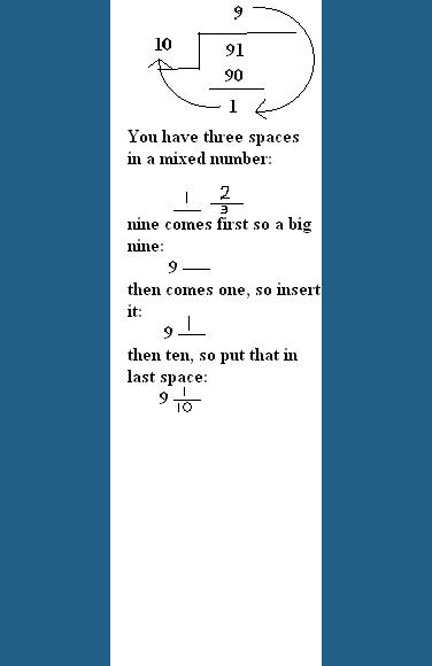# How To Find The Mixed Number And Decimal For Nine And One Tenth?

Shade nine grids of ten equal parts and one grid of ten equal parts.
thanked the writer.

9 1/10 as a mixed number will be 91/10

What you do is you multiply the denominator by the whole number, then you add the numerator.

9 1/10 as a decimal is 9.1

thanked the writer.
Well, a nine and one tenth means nine added to one-tenth. Now, since one tenth means 1/10, it equals to 0.1, which makes nine and one tenth=9.1. If your son has problem, just tell him to add 9 and 1/10 by taking the LCM. He will eventually end up with 91/10. Now tell him to divide it and stop where the first remainder comes. The way I learned to make mixed numbers seems the best to me. Tell your son to see how the clock arm turns and follow the direction to make a mixed number after he's done with division.(See the picture and you will understand it. I taught this to many juniors and they find it pretty simple to learn. I hope your child does too)